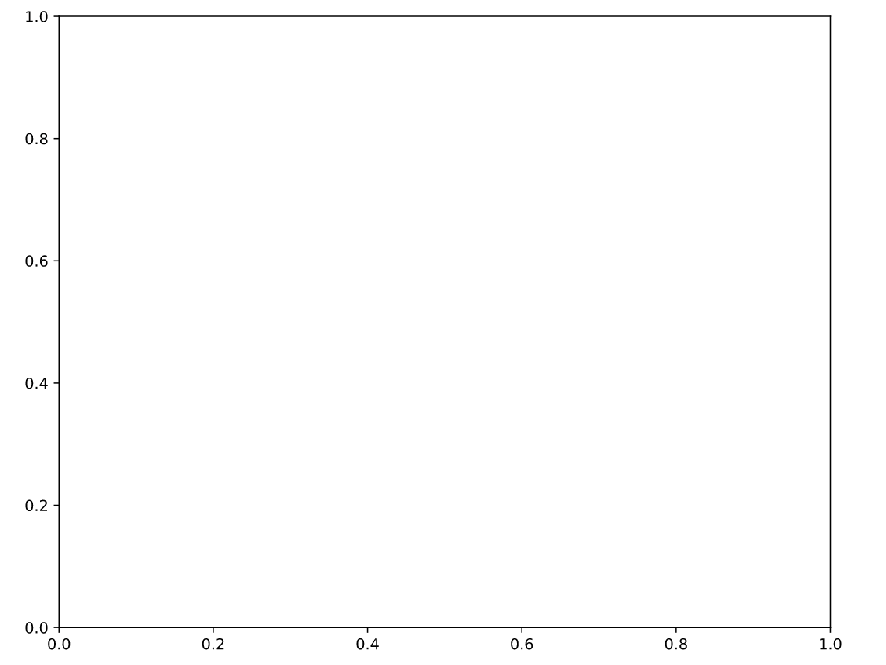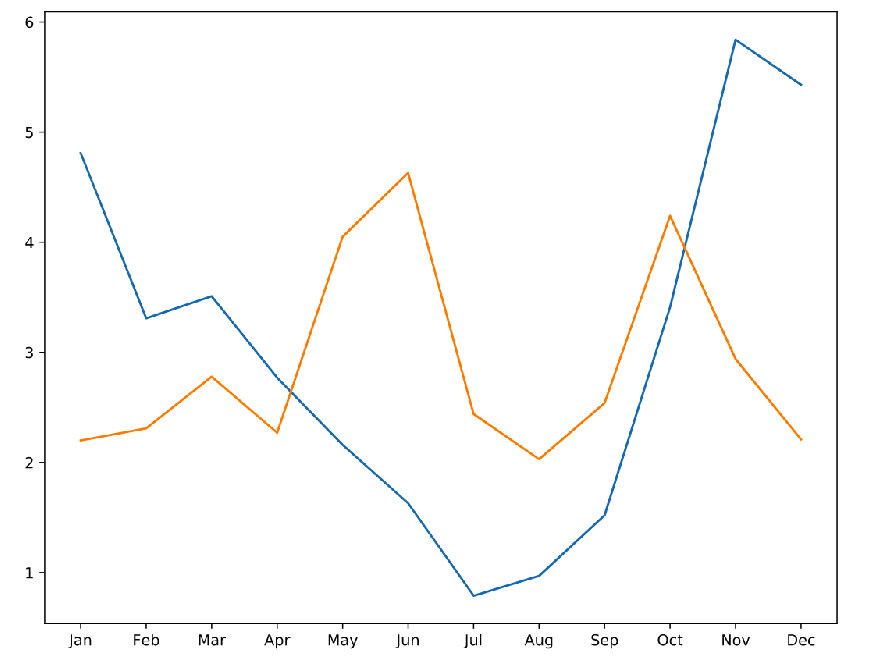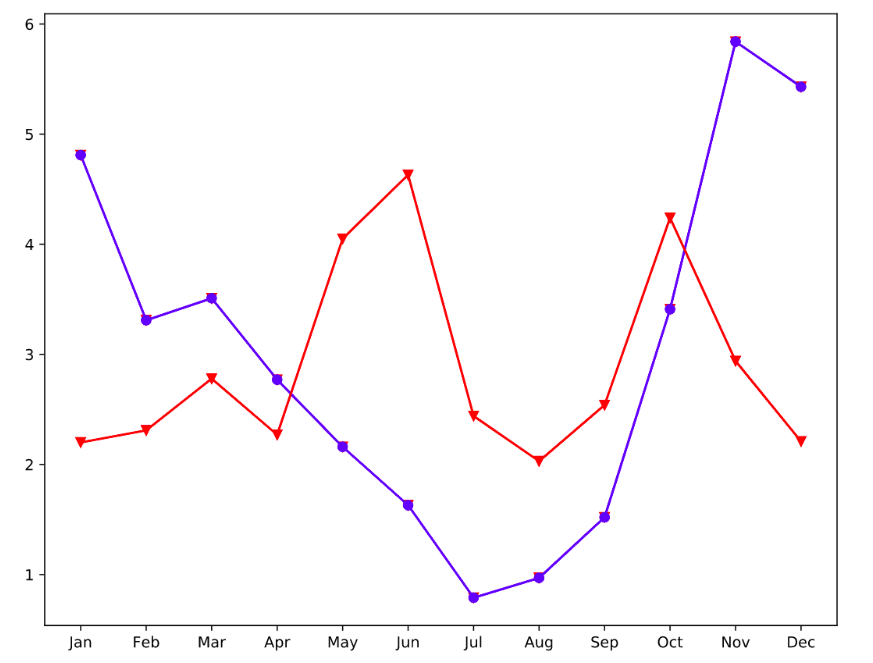# Data Visualization with Matplotlib in Python (Part 1)So here is my first blog regarding the data visualization with matplotlib in python. In this article we will cover the basic of the visualization with matplotlib.

So firstly let’s understand the visualization first .

Human mostly understand things by seeing instead of reading. “A Picture depicts thousand of words. A Large amount of Data which cannot be depict through large set of texts and tabular data can easily be understand through visual.Data visualization derives insights from the data.”

# 1. Introduction to the Pyplot interface:

There are many ways to use to Matplotlib. In this article I used the Pyplot interface.

``````#Import the matplotlib.pyplot submodule and name it plt
import matplotlib.pyplot as plt

#Create a Figure and an Axes with plt.subplots
fig, ax = plt.subplots()
# Call the show function
plt.show()``````

The plt.subplots() function when invoked without the inputs , it create two different objects:

1. fig -> fig object is just like container that holds things you see on the page.
2. ax -> axes object holds the data . To understand better , its just a canvas on which we can draw with our data to visualize it.

When you hit this code you can see the figure with the empty axis as shown in fig (1).Fig (1)

Let’s see it with the data . So we can use any basic datasets to know about the plots, I used my own so its your choice to take any data for the practice purpose.

So here I have datasets of the weather , in which its shows the precipitation according to the months from JAN to DEC.

``````#Create a Figure and an Axes with plt.subplots
fig, ax = plt.subplots()

# Plot PRECIPITATION_NML from north_weather against the MONTH
ax.plot(north_weather[“MONTH”], north_weather[‘PRECIPITATION_NML’])
# Plot PRECIPITATION_NML from south_weather against MONTH
ax.plot(south_weather[“MONTH”], south_weather[‘PRECIPITATION_NML’])
#Call the show function
plt.show()``````

So here I used two different plot in same figure and output would be two line plot in single graph. We also use the single plot axis, I used two axis here. Its your choice, how you want to visualize data. Let hit the show() function and output will be like as shown in fig(2).fig (2)

# 2. Customizing the Plots:

Let’s we customize plot according to our needs. We can do many customization in plots like change the colorlines etc.

2.1 Coloring and Markers :

``````# Plot North data
ax.plot(north_weather[“MONTH”], north_weather[“PRECIPITATION_NML”], color = ‘b’,marker = ‘o’)

# Plot South data
ax.plot(south_weather[“MONTH”], south_weather[“PRECIPITATION_NML”],color = ‘r’,marker = ‘v’ )
# Call show to display the resulting plot
plt.show()``````

In this code snippet we changed the color with color argument and marker . We can see the output in the fig(3).fig (3)

2.2 Labeling :

To make our plot more easily understandable we will use the the set__xlabel_() and set_ylabel() function and will also give the title to our plot with help of _set_title() _function. Let us have a look on code snippet. The fig(4) shows the output of the following snippet.

## How To Build A Data Science Career In 2021

In Conversation With Dr Suman Sanyal, NIIT University,he shares his insights on how universities can contribute to this highly promising sector and what aspirants can do to build a successful data science career.

## How To Blend Data in Google Data Studio For Better Data Analysis

Google Data Studio helps us understand the meaning behind data, enabling us to build beautiful visualizations and dashboards that transform data into stories.

## Exploratory Data Analysis is a significant part of Data Science

Data science is omnipresent to advanced statistical and machine learning methods. For whatever length of time that there is data to analyse, the need to investigate is obvious.